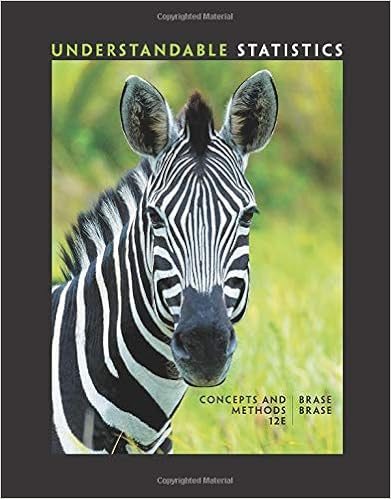# Two points ten peas are generated from parents having

• Test Prep
• 6
• 96% (24) 23 out of 24 people found this document helpful

This preview shows page 4 - 6 out of 6 pages.

##### We have textbook solutions for you!
The document you are viewing contains questions related to this textbook.The document you are viewing contains questions related to this textbook.
Chapter 3 / Exercise 2
Understandable Statistics: Concepts and Methods
BraseExpert Verified
9. (two points) Ten peas are generated from parents having the green/yellow pair of genes so there is a 75% probability that an individual pea will have a green pod. Find the probability that among the 10 offspring peas, at least 7 have green pods.
##### We have textbook solutions for you!
The document you are viewing contains questions related to this textbook.The document you are viewing contains questions related to this textbook.
Chapter 3 / Exercise 2
Understandable Statistics: Concepts and Methods
BraseExpert Verified
10. (two points) I always wanted to give away my vast wealth, so I am going to have a lottery next year. You have to pick the five winning numbers from 1-20. Each randomly selected numberis different and order doesn’t matter. What is the probability of your winning?
11. (two points) In a study of families with children with disabilities, group of 6 US households were randomly selected. In the table below, the random variable x represents the number of households among 6 that had a child with a disability living there. xP(x)00.0210.1520.2930.3140.1150.12a)Is this a probability distribution? b)What is the mean?c)What is the standard deviation?12. (two points) I am going to roll two fair dice. a)List the sample space. 36
b)What is the probability that I will roll a 3 on one of them when I roll both at the same time?
c)What is the probability that I will roll at least a sum of 7 when I roll them both?
d)Define mutually exclusive.
e)Define independent.
•••